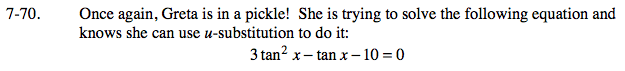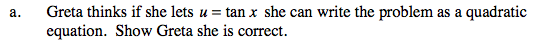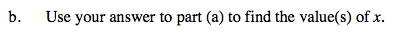### Home > CALC > Chapter 7 > Lesson 7.2.2 > Problem7-70

7-70.
1. Once again, Greta is in a pickle! She is trying to solve the following equation and knows she can use u-substitution to do it: Homework Help ✎

3 tan2 x − tan x − 10 = 0

1. Greta thinks if she lets u = tan x she can write the problem as a quadratic equation. Show Greta she is correct.

2. Use your answer to part (a) to find the value(s) of x.3u2u − 10 = 0
Solve for u.
Then solve for x.x = tan−1(2) ≈ 1.107 + πn